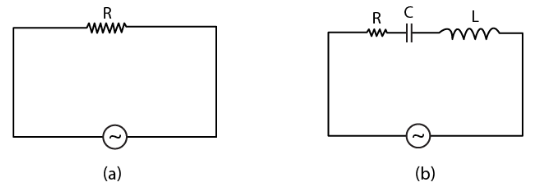# Study the circuits (a) and (b) shown`
Question:

Study the circuits (a) and (b) shown in Fig 7.2 and answer the following questions.(a) Under which conditions would the rms currents in the two circuits be the same?

(b) Can the rms current in the circuit (b) be larger than that in (a)?

Solution:

(a) (Irms)a = (Irms)b

Xl = Xc

R = √R2 + (Xl – Xc)2

Therefore, Irms will be equal in (a) as well (b) if Xl = Xc

(b) For (Irms)b > (Irms)a

√R2 + (Xl – Xc)< R

(Xl < Xc)2 < 0

Reactance cannot be negative.